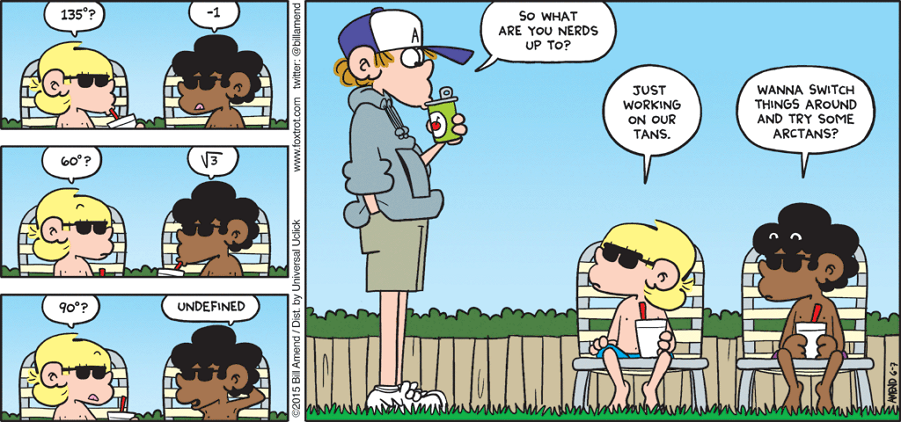# Need some tanning$\large \tan(63^\circ) = \sqrt{\sqrt a-\sqrt b} + \sqrt{\sqrt c-\sqrt b}$

The equation above is true for positive integers $a,b,$ and $c.$ What is the value of $a+b+c?$

×